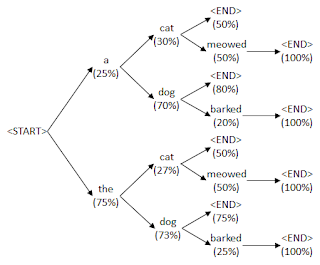## Sunday, October 30, 2016

### Using beam search to generate the most probable sentence

This blog post continues in a second blog post about how to generate the top n most probable sentences.

In my last blog post I talked about how to generate random text using a language model that gives the probability of a particular word following a prefix of a sentence. For example, given the prefix "The dog", a language model might tell you that "barked" has a 5% chance of being the next word whilst "meowed" has a 0.3%. It's one thing generating random text in a way that is guided by the probabilities of the words but it is an entirely different thing to generate the most probable text according to the probabilities. By most probable text we mean that if you multiply the probabilities of all the words in the sentence together you get the maximum product. This is useful for conditioned language models which give you different probabilities depending on some extra input, such as an image description generator which accepts an image apart from the prefix and returns probabilities for the next word depending on what's in the image. In this case we'd like to find the most probable description for a particular image.

You might think that the solution is to always pick the most probable word and add it to the prefix. This is called a greedy search and is known to not give the optimal solution. The reason is because it might be the case that every combination of words following the best first word might not be as good as those following the second best word. We need to use a more exploratory search than greedy search. We can do this by thinking of the problem as searching a probability tree like this:The tree shows a probability tree of which words can follow a sequence of words together with their probabilities. To find the probability of a sentence you multiply every probability in the sentence's path from <start> to <end>. For example, the sentence "the dog barked" has a probability of 75% × 73% × 25% × 100% = 13.7%. The problem we want to solve is how to find the sentence that has the highest probability.

One way to do this is to use a breadth first search. Starting from the <start> node, go through every node connected to it, then to every node connected to those nodes and so on. Each node represents a prefix of a sentence. For each prefix compute its probability, which is the product of all the probabilities on its path from the <start> node. As soon as the most probable prefix found is a complete sentence, that would be the most probable sentence. The reason why no other less probable prefixes could ever result in more probable sentences is because as a prefix grows, its probability shrinks. This is because any additional multiplications with probabilities made to any prefix probability will only make it smaller. For example, if a prefix has a probability of 20% and another word is added to it which has a probability of 99%, then the new probability will be 20% × 99% which is the smaller probability of 19.8%.

Of course a breadth first search is impractical on any language model that has a realistic vocabulary and sentence length since it would take too long to check all the prefixes in the tree. We can instead opt to take a more approximate approach where we only check a subset of the prefixes. The subset would be the top 10 most probable prefixes found at that point. We do a breadth first search as explained before but this time only the top 10 most probable prefixes are kept and we stop when the most probable prefix in these top 10 prefixes is a complete sentence.

This is practical but it's important that the way we find the top 10 prefixes is fast. We can't sort all the prefixes and choose the first 10 as there would be too many. We can instead use a heap data structure. This data structure is designed to quickly take in a bunch of numbers and quickly pop out the smallest number. With this you can insert the prefix probabilities one by one until there are 10 prefixes in it. After that start popping out the smallest probability immediately after inserting a new one in order to only keep the 10 largest ones.

Python provides a library called "heapq" (heap queue) just for this situation. Here is a class that makes use of heapq in order to create a beam of prefix probabilities:

import heapq

class Beam(object):
#For comparison of prefixes, the tuple (prefix_probability, complete_sentence) is used.
#This is so that if two prefixes have equal probabilities then a complete sentence is preferred over an incomplete one since (0.5, False) < (0.5, True)

def __init__(self, beam_width):
self.heap = list()
self.beam_width = beam_width

heapq.heappush(self.heap, (prob, complete, prefix))
if len(self.heap) > self.beam_width:
heapq.heappop(self.heap)

def __iter__(self):
return iter(self.heap)


The code to perform the actual beam search is this:

def beamsearch(probabilities_function, beam_width=10, clip_len=-1):
prev_beam = Beam(beam_width)
while True:
curr_beam = Beam(beam_width)

#Add complete sentences that do not yet have the best probability to the current beam, the rest prepare to add more words to them.
for (prefix_prob, complete, prefix) in prev_beam:
if complete == True:
else:
#Get probability of each possible next word for the incomplete prefix.
for (next_prob, next_word) in probabilities_function(prefix):
if next_word == '<end>': #if next word is the end token then mark prefix as complete and leave out the end token